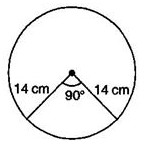Courses

# Test: Area Related To Circles- 3

## 25 Questions MCQ Test Mathematics (Maths) Class 10 | Test: Area Related To Circles- 3

Description
This mock test of Test: Area Related To Circles- 3 for Class 10 helps you for every Class 10 entrance exam. This contains 25 Multiple Choice Questions for Class 10 Test: Area Related To Circles- 3 (mcq) to study with solutions a complete question bank. The solved questions answers in this Test: Area Related To Circles- 3 quiz give you a good mix of easy questions and tough questions. Class 10 students definitely take this Test: Area Related To Circles- 3 exercise for a better result in the exam. You can find other Test: Area Related To Circles- 3 extra questions, long questions & short questions for Class 10 on EduRev as well by searching above.
QUESTION: 1

### The area of a circle is 38.5 sq. cm. Its circumference is

Solution:

Given: Area of the circle = πr2 - 38 5 sq. cm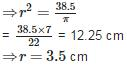∴ Circumference of the circle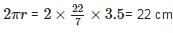QUESTION: 2

### The area of the square that can be inscribed in a circle of radius 12 cm is

Solution: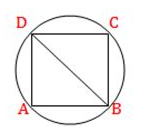According to question, Diameter (BD) = 2 x radius = 2 x 12 = 24 cm
∴ Area of square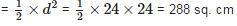QUESTION: 3

### If the area of a circle is equal to the area of a square, then the ratio of their perimeters is

Solution:

Let the radius of the circle be rr and the side of the square be a.a. Then according to the question,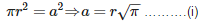Now, Ratio of their perimeters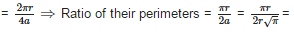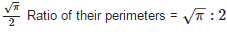QUESTION: 4

A piece of wire 20cm long is bent into the form of an arc of a circle subtending an angle of 60 at its centre. The radius of the circle is

Solution: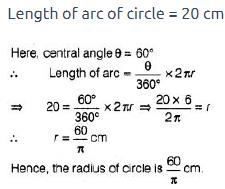QUESTION: 5

The area of a sector of a circle with radius 21cm and sector angle 120 is

Solution:

Area of the sector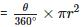⇒ Area of the sector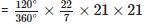= 462 sq. cm

QUESTION: 6

The circumference of a circle whose diameter is 4.2cm is

Solution:

Given : Diameter (d) = 4.2 cm
∴ Circumference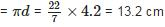QUESTION: 7

The area of the circle that can be inscribed in a square of side 10cm is

Solution: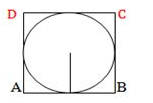Since if a circle inscribed a square. then the radius of the circle is half of to side of square.
∴  Radius = 10/2 = 5 cm
∴ Area of the circle = πr2 = π(5)2 = 25π sq. cm

QUESTION: 8

The area of a square that can be inscribed in a circle of radius 10cm is

Solution: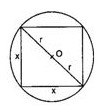Given: Radius (r) = 10 cm
Let side of the square be x cm
Now. using Pythagoras theorem.
x+ x2 = (2r)⇒ 2x2 = (20)2
⇒ 2x2 = 400 ⇒ x2 = 200 sq. cm
Therefore. area of the square = 200 sq. cm.

QUESTION: 9

A square of side 7cmis inscribed in a circle. The area enclosed between the circle and the square is

Solution: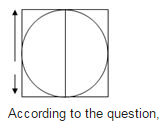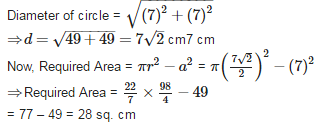QUESTION: 10

The angle described b the minute hand between 4.00 pm and 4.25 pm is

Solution:

Time duration between 4.00 om and 4.25 pm = 25 minutes
∵ Angle described by minute hand in 60 minutes = 360°
∴ Angle described by minute hand in 25 minutes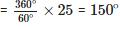QUESTION: 11

The length of the wire is 66m. The number of circles of circumference 13.2cm can be made from the wire is

Solution:

Given: Length of wire = 66 m = 6600 cm and Circumference = 13.2 cm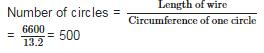QUESTION: 12

If the perimeter of a circle is equal to that of a square, then the ratio of their areas is

Solution:

Let the radius of the circle be rr and side of the square be a. Then, according to question,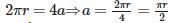Now, ratio of their areas,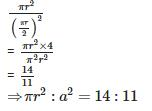QUESTION: 13

If the area and circumference of a circle are numerically equal, then its radius is

Solution:

Let radius of the circle berunits.
∴ Area of the circle = Circumference of the circle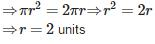QUESTION: 14

On a square handkerchief, nine circular designs each of radius 7cmare made. The area of the remaining portion of the handkerchief is

Solution:

Here Side of square ABCD = AB = 3 x diameter of circular design
⇒ AB = 3 x (2 x 7) = 42 cm
∴ Area of square = 42 x 42 = 1764 cm2
And Area of one circular design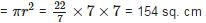And Area of one circular design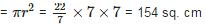∴ Area of 9 circular designs = 154 x 9 = 1386 sq. cm
∴ Area of remaining portion of handkerchief = 1764 - 1386 = 378 sq. cm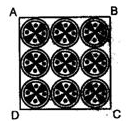QUESTION: 15

A horse is tied to a peg at one corner of a square shaped gross field of side 25m by means of a 14m long rope. The area of that part of the field in which the horse can graze is

Solution: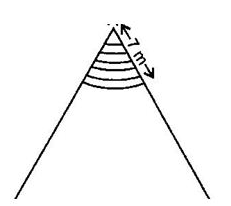Area of the shaded region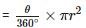Area of the shaded region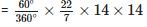Area of the shaded region = 154 sq. cm

QUESTION: 16

The perimeter of a protractor is

Solution:

Let radius of the protractor be r
∴ Perimeter of protractor = Perimeter of semicircle + Diameter of semicircle ⇒ Perimeter of protractor = πr+2r

QUESTION: 17

The radius of a circle whose circumference is equal to the sum of the circumferences of the two circles of diameters 36cm and 20cm is

Solution:

Let required radius be R. Then according to the question,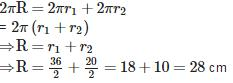QUESTION: 18

If the radius of a circle is increased by 100%, then its area is increased by

Solution:

Area of the circle with radius r =πr2
Now, New Radius = r + 100% of r = r + r = 2r
∴ New Area = π(2r)2 - 4πr2
∴ Increased Area = 4πr2 -  πr2 = 3πr2
And Area increased in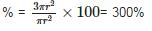QUESTION: 19

If the area of a circle is ‘A’, radius of the circle is ‘r’ and its circumference is ‘C’, then

Solution:

Here, Area of circle (A) = πr2 and Circumference of circle (C) = 2πr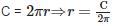Putting value of C in A = πr2, we get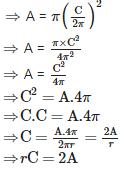QUESTION: 20

A light house throws light forming sector of radius 21 m with central angle 120. The area covered by it is

Solution:

Area of the sector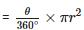⇒ Area of the sector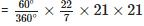⇒ Area of the shaded region = 462 sq. cm

QUESTION: 21

The circumference of a circle exceeds its diameter by 120cm, then its radius is

Solution: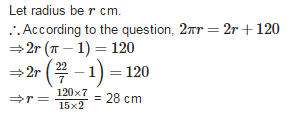QUESTION: 22

If the circumference of a circle and the perimeter of a square are equal, then

Solution:

Let the radius of the circle be r and side of the square be a.
Then, according to question,
2πr = 4a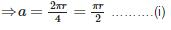Now, ratio of their areas,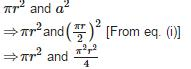Therefore, Area of the circle > Area of the square

QUESTION: 23

The distance around the circle is called its

Solution:

The distance around the circle is called its circumference

QUESTION: 24

The area of a quadrant of a circle whose circumference is 88cm is

Solution: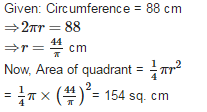QUESTION: 25

If a chord of a circle of radius14cm subtends a right angle at the centre of the circle, then the area of the sector is

Solution: